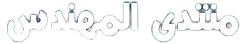# طلب مساعدتكم لا حرمكم الله الاجر

اخواني الأعزاء ،

انا طالب ابحث عن من يساعدني في مشروع صغير و نهاية تسليمه بعد اسبوعين

المشروع عبارة عن كتابة بعض الاكواد الصغيره في الماتلاب

وهنا اضع بين يديكم المشروع كاملا

[

1A: A prime number § is an integer that has no factors or divisors except 1 and itself. The first 12 prime numbers are 2,3,5,7,11,13,17,19,23,29,31, and 37. Note that 2 is the only even prime number. Prime numbers play a significant role in coding and computation algorithms and circuits.
A: Write a MATLAB code to find whether a given integer “x” is a prime.
B: Write a MATLAB code to find the number of primes less than or equal to a given real number “x” (not necessarily an integer). This is called “prime counting function, π(x)”. For example, π(10) = π(10.9) = 4.
C: Prime Number Theorem: As x becomes large, π(x) approaches x / ln(x). Prove using MATLAB simulation.

1B: Non-linear equations: Using MATLAB, find whether the equation cos(x) = x2 has a solution over the interval [-3,+3].

1C: 3D Plotting: Plot the function z(x,y) = cos(x)exp(-|y|) and its first derivatives. Plot a few 2D cross-sections of z.

1D: Computation algorithms are of fundamental importance in designing new generations of faster and more efficient computers and digital signal processors (DSP). Mersenne primes (MP’s) are important in computation algorithms and hardware as they enable binary arithmetic while using “Number Theoretic Transforms”. An integer m is a Mersenne Prime if it is a prime number of the form m = 2p - 1, where p is a prime. There are only 44 MP’s discovered so far. The first 9 MP’s are 3, 7, 31, 127, 8191, 131071, 524287, 2147483647, 2305843009213693951 (corresponding to p = 2, 3, 5, 7, 13, 17, 19, 31, 61), while the last known MP is m44=232582657 - 1 (discovered in 2006 and is composed of 9808358 digits). Write a MATLAB code to find the first 5 MP’s.

]

ارجوا ان اجد من يساعدني ولكم جزيل الشكر

حرام و الله عليكم

``````function []=detemine(x)
a=isprime(x)
if a==0
disp('it is not prime')
else
disp('it is prime')
end
end
``````
``````function [n]=number of primes(x)
n=0;
x=ceil(x);
for i=1:1:x
a=isprime(i);
if a==1
n=n+1
end
end
end
``````
``````[n]=numberof primes(x)
m=x/log(x)

``````